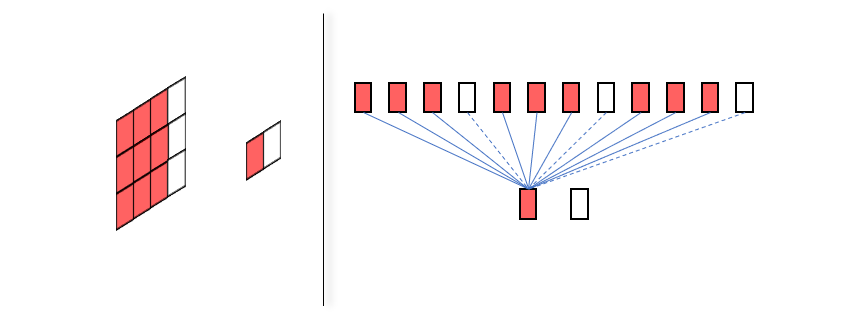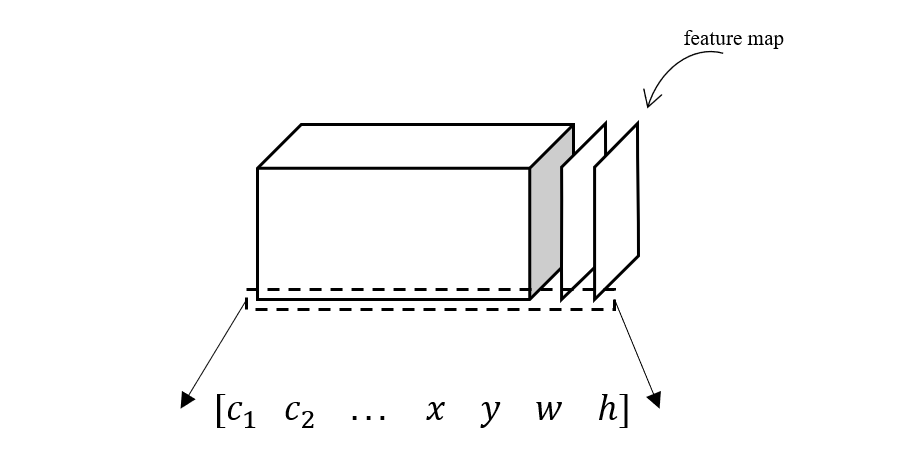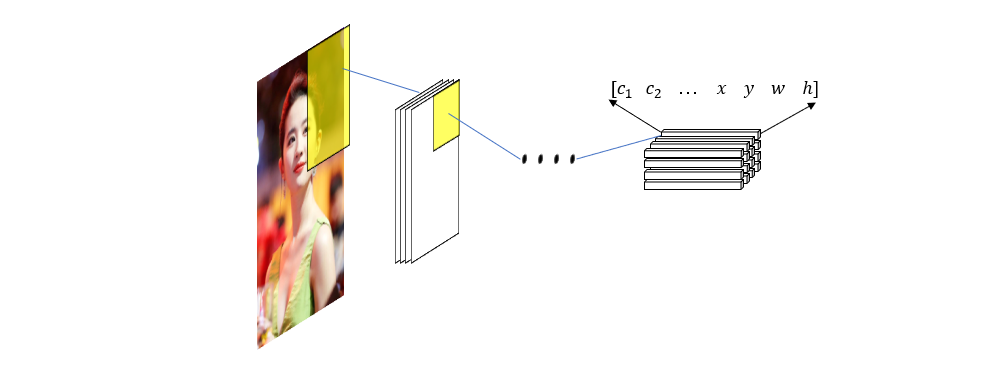Fenrier Lab

# 使用 PyTorch 实现 yolov3 算法 (1)

$[x, y, w, h]$

$[c_1, c_2, c_3, ... c_n, x, y, w, h]$

\left[ \begin{aligned} c_1^1 &\quad c_2^1&\quad ... &\quad x^1 \quad y^1 \quad w^1 \quad h^1\\ c_1^2 &\quad c_2^2&\quad ... &\quad x^2 \quad y^1 \quad w^1 \quad h^1\\ ...\\ c_1^n &\quad c_2^n&\quad ... &\quad x^n \quad y^n \quad w^1 \quad h^1 \end{aligned} \right]